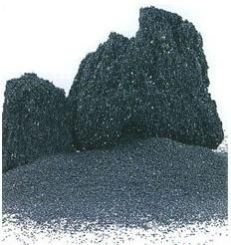JAGGO OVERSEAS

Delhi, India

Green Silicon Carbide• Weight : >= 98.50 <=0.20

 Chemical Composition Chemical composition (% by Weight) Grit Size Sic F.C Fe2O3 F12-F90 >= 98.50 <=0.20 <=0.60 F100-F150 >= 98.00 <=0.30 <=0.80 F180-F220 >=97.00 <=0.30 <=1.20

Looking for Green Silicon Carbide?